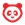# Community

cancel
Showing results for
Show  only  | Search instead for
Did you mean:

# New Quizzes Formula Question / Non numeric

I would like to see a formula question where the variable values can be generated by a formula, and does not necessarily have to be numeric.  Likewise, answers do not necessarily have to be numeric.  As an example take a question where a student is being asked to convert a hexadecimal number to binary. In this case "convert hexadecimal `x` to binary".  In this case I need to be able to write a formula to create possible values for `x`, and that formula needs to be able to generate non-numeric string values like "3BF" or "9A7".

Likewise if I asked a student to 'convert binary `y` to hexadecimal", I need to be able to write a formula which will generate a random string of 0s and 1s as possible values for `y` (such as 00110), and I also need the student to be able to enter a non-numeric answer, such as "3BF" or "9A7".Community Team
Status changed to: OpenCommunity Member

A formula for generating the problem values shown in the "Question" would be valuable.  Even something like having students divide and end up with integer answers can't be done as a formula question.  If I could enter the problem in the "Question" as `a*b`/`b` and then set the value ranges for a and b, it would work.

Sometimes I just want odd or even numbers in the problem.  I can't do that currently.  I would love to enter formulas like `2n` or `2n+1`.

Instead of a formula in the "Question", we could define variables in the value ranges area.  Instead of only doing a range from low to high, we could choose between range, list, or formula.  For formula, we could have a variable defined by the other variables.  So,

in the "Question", we would have `n`/`b`.

in the value ranges area, we would have

1. n=(formula) a*b
2. a=(range) Min 1 Max 10
3. b=(list) 1, 2, 3, 5, 7

Combined with the following suggestions and "Formula Question" would be a quiz option of superhero levels of power.

Variables used by multiple questions: https://community.canvaslms.com/t5/Idea-Conversations/Grouped-Formula-Questions-Shared-Set-of-Define...

Random Value Selection Options:  https://community.canvaslms.com/t5/Idea-Conversations/New-Quizzes-Formula-Question-Pick-from-set/idi...Community Member

Another thought:

What if we randomly select the answer and put it through a series of steps to create the problem the student is presented with?

In the "Question" we enter `a`.

In the value range, we enter

1. x= Min 1 Max 10
2. f= Min 1 Max 10
3. g= Min 1 Max 10
4. h=x*f+g
5. a= " `f`x + `g`=`h` "

If the formula picks x=3, f=2, and g=1, the student gets 2x+1=7.  The answer of 3 is guaranteed to come out as intended because the formula started with the answer to create the problem.New Member

Hi Canvas,

One feature that would be very helpful would be the ability to use formulas (like the formula questions) but to have a multiple choice answer format.

For example:

Question:

A person walks to the store traveling at {3 m/s} for {7.2 s}. How far did the person travel during the {7.2 s}?

The 3m/s and 7.2 s are variables with defined ranges and decimals.

The formula: answer = {3 m/s}*{7.2 s}

formula: distractor1 = answer * {0.3}     (0.3 is a variable with a defined range)

formula: distractor2 = answer * {1.9}       (1.9 is a variable with a defined range)

formula: distractor 3 = answer + {2.4}       (2.4 is a variable with a defined range)

Student view:

A person walks to the store traveling at 3 m/s for 7.2 s. How far did the person travel during the 7.2 s?

A) 21.6 m

B) 6.5 m

C) 41.0 m

D) 24 m

Students look to see if their answer of 21.6 m is an option.

This type of question format would allows students to check their answer with the possible answer choices to see if any of them match what they did. If one matches, it gives the student confidence that they did the calculations correctly. If none of the answers match, the student knows to recheck their work for a possible numerical error or conceptual one.

This also allows the students to transition into the harder, formula based questions with an numerical response.

Furthermore, with the formulas, each student can have their own dynamically created question so that students can't share numerical answers.

I think this type of option would benefit a lot of math and mathematical science classes.

Thanks,

Sean